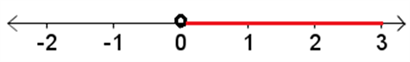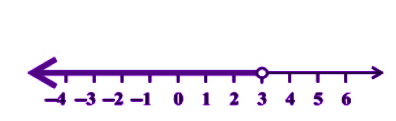Checkout JEE MAINS 2022 Question Paper Analysis : Checkout JEE MAINS 2022 Question Paper Analysis :

# Represent Linear Inequalities In One Variable On Number Line

To learn how to represent the linear inequalities in one variable on the number a line, we have created this article. Basically, in linear inequalities instead of using equal to a symbol (=), we use greater than (>), less than (<), greater than or equal (≥) and less than or equal (≤) symbols. ‘Equal to’ symbol is used for linear equalities or show equalities between any two expression or numbers.

Linear Inequalities Definition: Any two real numbers or two algebraic expressions equated by the symbol ‘<’, ‘>’, ‘≤’ or ‘≥’ form an inequality. For example, 6<8, 5>2 are examples of numerical inequalities. and x<7, y >10, x ≥ y > 9 are the examples of algebraic or literal inequalities.

The symbols ‘<‘ and ‘>’ represent the strict inequalities and the symbols ‘≤’ and ‘≥’ represent slack inequalities. The examples like mx+n>0, mx+n<0, mx + n ≤ 0 and mx + n ≥ 0 are the linear inequalities in one variable. Now, let us learn how to specify them in a number line.

## Algebraic Solutions of Linear Inequalities in One Variable

We know different types of linear inequalities. Let’s learn how to solve the inequalities.

For example: Consider the linear inequality given below;

3x < 6, where x is a whole number

LHS of the inequality is 3x and RHS of the inequality is 6

When x = 0,

LHS = 3 × 0 = 0, RHS = 6

Since 0 is less than 6, x = 0 satisfies the inequality.

When x = 1,

LHS = 3 × 1 = 3, RHS = 6

Since 3 is less than 6, x = 1 satisfies the inequality.

When x = 2,

LHS = 3 × 2 = 6, RHS = 6

Since 6 is not less than 6, x = 2 will not satisfy the inequality.

It is understood that x should be less than 2 to satisfy the inequality.

“Values of the variable which make the statement true are called solutions of the inequality”.

Therefore, solutions of the above inequality are 0 and 1 and {0, 1} is called the solution set.

In the above example, we have got the solutions by trial and error method which is not always effective. It becomes time-consuming when the numbers are big and there are many conditions.There are two rules to be followed while solving inequalities. That will be explained with the following example.

### Rules for Linear Inequalities

Rule 1: Equal numbers can be added to or subtracted from both the sides of the inequality without changing the sign of inequality.

Rule 2: Same positive number can be multiplied or divided into both sides of the inequality. Sign of inequality is reversed if both sides are multiplied or divided by a negative number.

### Example of Algebraic Solutions of Linear Inequality

Let us solve some examples on linear inequalities in one variable

Example 1: 3 – 2x < 1 , solve for x if x is an integer less than 6.

3 – 2x < 1

Subtract 3 from both the sides,

3 – 3 – 2x < 1 – 3

-2x < -2

Dividing both sides of the inequality by -2,

(Note that -2 is a negative number, a sign of the inequality has to be reversed)

-2x/-2 > -2/-2

x > 1

Since x is an integer less than 6, solution set of x is {2, 3, 4, 5}

Let’s take another example and represent the solution of inequality on a number line.

### Representation of Linear Inequalities On Number Line

Let us solve some examples on linear inequalities in one variable to show on a number line.

Example 2: Solve 2x + 3 ≤ 9 , represent ‘x’ on a number line if x is positive.

2x + 3 ≤ 9

Subtract 3 from both sides,

2x + 3 – 3 ≤ 9 – 3

2x ≤ 6

Dividing both sides by 2 gives,

2x/2 ≤ 6/2

x ≤ 3

It is given that x is positive.

Therefore x is greater than zero and less than or equal to 3. i.e.,x ∈(0, 3]Example 3: Solve 6x + 2 < 4x + 8. Show the graph of the solutions on a number line.

Solution: 6x + 2 < 4x + 8.

2x < 6

x < 3

The graphical representation will be:### Linear Inequalities in One Variable Word Problems

Example 4: The marks scored by a student of Class 10th in the first and second terminal examination are 60 and 45, respectively. Find the minimum marks he should get in the annual examination to have an average of at least 62 marks.

Solution: Let x be the marks obtained by the student in the annual examination. Then;

(60 + 45 + x)/3 ≥ 62
or 105 + x ≥ 196
or x ≥ 93
Thus, the student must obtain a minimum of 93 marks to get an average of at least 62 marks in the annual exam.

Example 5: Find all pairs of consecutive odd natural numbers, both of which are larger than 10, such that their sum is less than 40.

Solution: Let x be the smaller of the two consecutive odd natural number so that the other one is x +2. Then, we should have:

x > 10 ………….(1)

and

x + ( x + 2) < 40 ………..(2)

On solving the above equation, we get;

2x + 2 < 40

x + 1< 20

x < 19 ………….(3)

From eq.1 and 3 we get:

10 < x < 19

Since x is an odd number, x can take the values 11, 13, 15, and 17.

So, the required possible pairs will be (11, 13), (13, 15), (15, 17), (17, 19)

Get more knowledge from BYJU’S about any topic along with linear equations and increase your knowledge level. Download BYJU’S-The Learning App to get for a personalised learning experience.